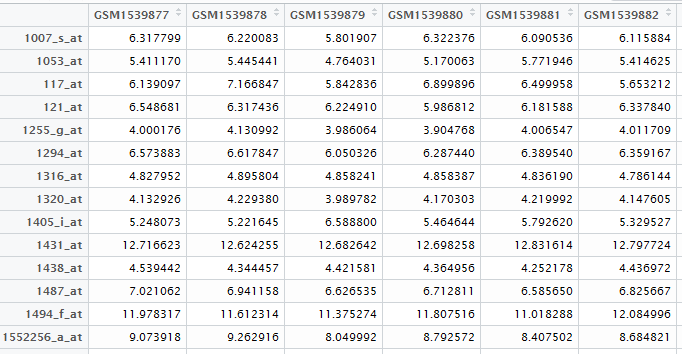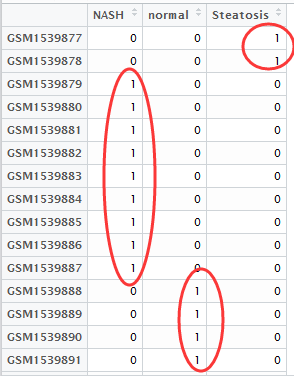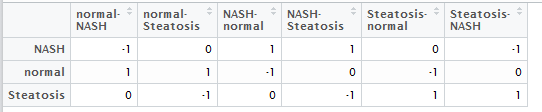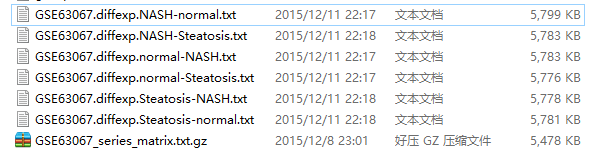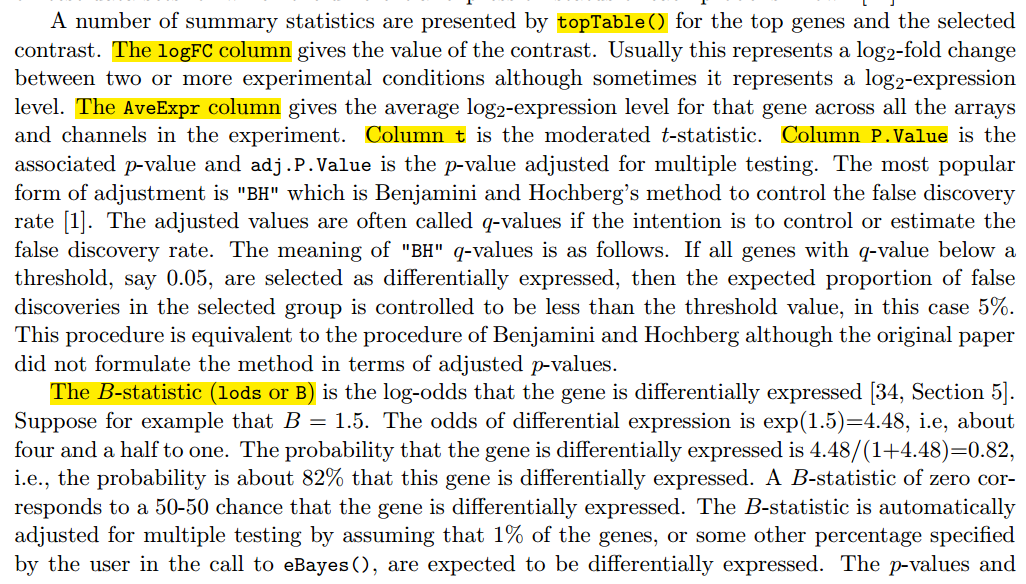01

01

05

11

# 用limma包的voom函数来对RNA-seq数据做差异分析

limma真不愧是最流行的差异分析包，十多年过去了，一直是芯片数据处理的好帮手。

09

# 差异分析是否需要比较矩阵

• 表达矩阵
• 分组矩阵
• 差异比较矩阵

## 大家仔细观察下面的两个代码

### 首先是不需要差异比较矩阵的

```    library(CLL)
data(sCLLex)
library(limma)
design=model.matrix(~factor(sCLLex\$Disease))
fit=lmFit(sCLLex,design)
fit=eBayes(fit)
options(digits = 4)
logFC AveExpr      t   P.Value adj.P.Val     B
39400_at  1.0285   5.621  5.836 8.341e-06   0.03344 3.234
36131_at -0.9888   9.954 -5.772 9.668e-06   0.03344 3.117
33791_at -1.8302   6.951 -5.736 1.049e-05   0.03344 3.052
1303_at   1.3836   4.463  5.732 1.060e-05   0.03344 3.044
36122_at -0.7801   7.260 -5.141 4.206e-05   0.10619 1.935
36939_at -2.5472   6.915 -5.038 5.362e-05   0.11283 1.737
41398_at  0.5187   7.602  4.879 7.824e-05   0.11520 1.428
32599_at  0.8544   5.746  4.859 8.207e-05   0.11520 1.389
36129_at  0.9161   8.209  4.859 8.212e-05   0.11520 1.389
37636_at -1.6868   5.697 -4.804 9.355e-05   0.11811 1.282
```

### 然后是需要差异比较矩阵的

```    library(CLL)
data(sCLLex)
library(limma)
design=model.matrix(~0+factor(sCLLex\$Disease))
colnames(design)=c('progres','stable')
fit=lmFit(sCLLex,design)
cont.matrix=makeContrasts('progres-stable',levels = design)
fit2=contrasts.fit(fit,cont.matrix)
fit2=eBayes(fit2)
options(digits = 4)

logFC AveExpr      t   P.Value adj.P.Val     B
39400_at -1.0285   5.621 -5.836 8.341e-06   0.03344 3.234
36131_at  0.9888   9.954  5.772 9.668e-06   0.03344 3.117
33791_at  1.8302   6.951  5.736 1.049e-05   0.03344 3.052
1303_at  -1.3836   4.463 -5.732 1.060e-05   0.03344 3.044
36122_at  0.7801   7.260  5.141 4.206e-05   0.10619 1.935
36939_at  2.5472   6.915  5.038 5.362e-05   0.11283 1.737
41398_at -0.5187   7.602 -4.879 7.824e-05   0.11520 1.428
32599_at -0.8544   5.746 -4.859 8.207e-05   0.11520 1.389
36129_at -0.9161   8.209 -4.859 8.212e-05   0.11520 1.389
37636_at  1.6868   5.697  4.804 9.355e-05   0.11811 1.282```

design=model.matrix(~factor(sCLLex\$Disease))

design=model.matrix(~0+factor(sCLLex\$Disease))

22

# 用RankComp的思想来做差异基因分析

Wang H, Sun Q, Zhao W, et al. Individual-level analysis of differential expression of genes and pathways for personalized medicine[J]. Bioinformatics, 2014: btu522.

> table(rank_comp)
rank_comp
down        no        up
58479 465752098     58479
>

# 用excel表格做差异分析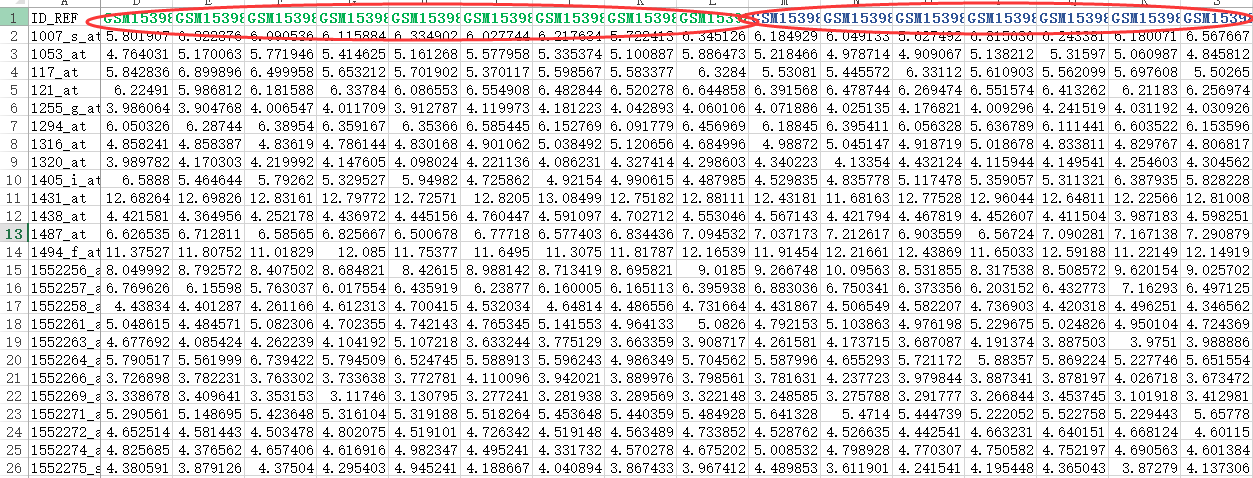=AVERAGE(D2:L2)    ##求NASH组的平均表达量

=AVERAGE(M2:S2)    ###求normal的平均表达量

=T2-U2             ##计算得到logFOLDchange值

=AVERAGE(D2:S2)    ###得到所有样本的平均表达量

=T.TEST(D2:L2,M2:T2,2,3)  ###用T检验得到两个组的表达量的差异显著程度。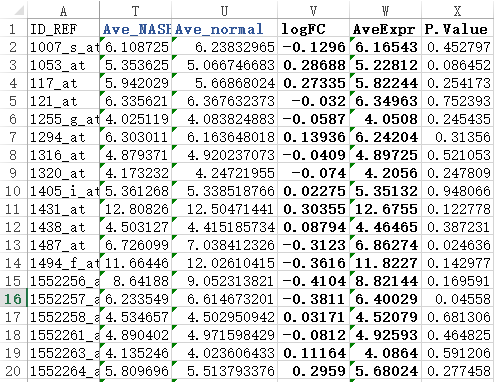# 用limma包对芯片数据做差异分析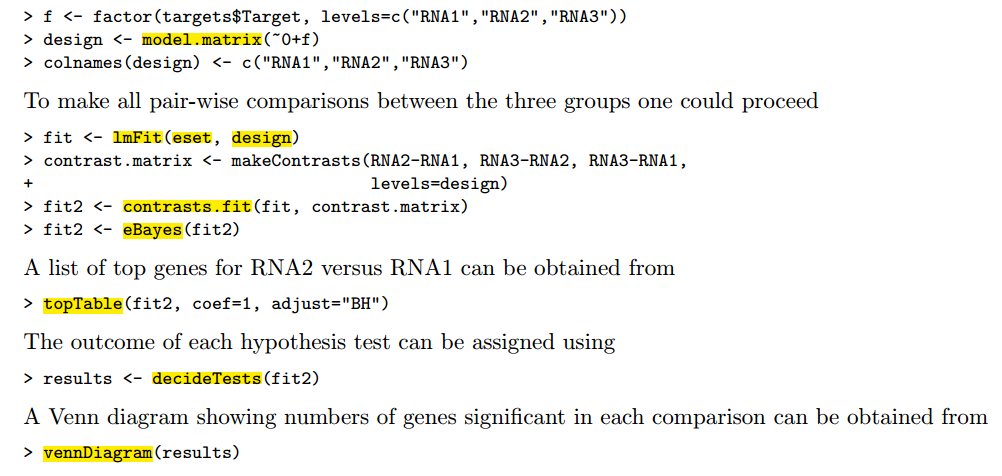rownames(exprSet)=exprSet[,1]

exprSet=exprSet[,-1]